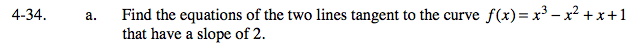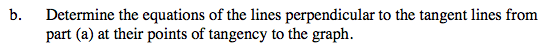### Home > CALC > Chapter 4 > Lesson 4.1.3 > Problem4-34

4-34.Set slope function equal to 2: f '(x) = 2 and solve for x. You should get two solutions.

Evaluate f(x) at both values of x. This will give you the two points of tangency.

Write the equation of the two tangent lines in point-slope form.Perpendicular lines have negative reciprocal slopes.

Use the eTool below to examine the graph.
Click the link at right for the full version of the eTool: Calc 4-34 HW eTool